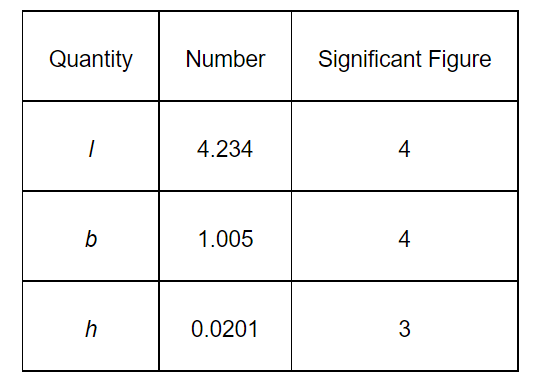# The length, breadth and thickness of a rectangular sheet of metal

Question.

The length, breadth and thickness of a rectangular sheet of metal are $4.234 \mathrm{~m}, 1.005 \mathrm{~m}$, and $2.01 \mathrm{~cm}$ respectively. Give the area and volume of the sheet to correct significant figures.

solution:

Length of sheet, $I=4.234 \mathrm{~m}$

Breadth of sheet, $b=1.005 \mathrm{~m}$

Thickness of sheet, $h=2.01 \mathrm{~cm}=0.0201 \mathrm{~m}$

The given table lists the respective significant figures:Hence, area and volume both must have least significant figures i.e., 3.

Surface area of the sheet $=2(l \times b+b \times h+h \times l)$

$=2(4.234 \times 1.005+1.005 \times 0.0201+0.0201 \times 4.234)$

$=2(4.25517+0.0202005+0.0851034)$

$=2 \times 4.36$

$=8.72 \mathrm{~m}^{2}$

Volume of the sheet = l × b × h

$=0.0855 \mathrm{~m}^{3}$

This number has only 3 significant figures i.e., 8, 5, and 5.